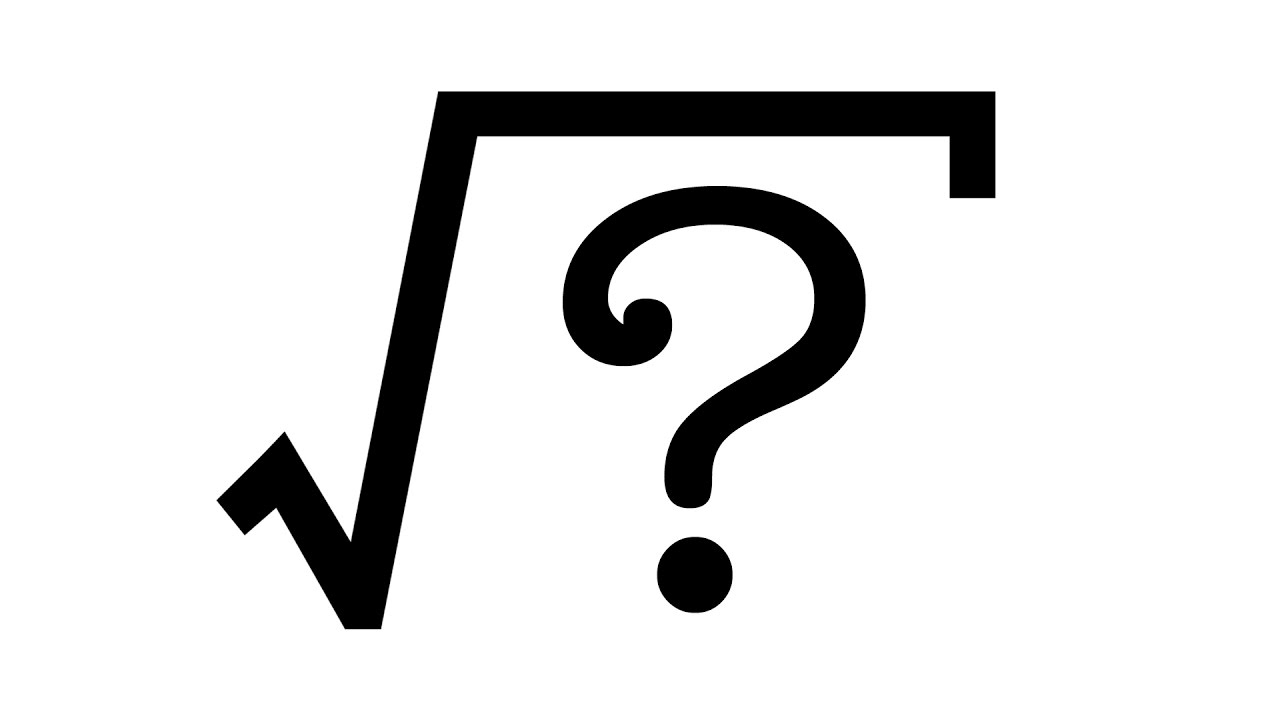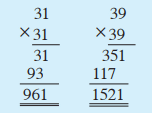# Find Square Root of a Number – Super Easy Tricks by Jason

No account yet? Register

Hi everyone! Some might see finding the square root of the given number as a bit hard. So here’s my post, which will make it super easy for you and will help you more than ever. Don’t miss this chance.

So by studying this post well, you will be able to,

•  Identify what a perfect square is
•  Write the square of each of the whole numbers from 1 to 20
•  Find the square root of the perfect squares from 1 to 1000 by observation and by considering their prime factors

### Square of a positive integer

A few numbers can be represented by a square-shaped arrangement of dots given below.

You have learned earlier that the numbers 1, 4, 9, 16, … which can be represented as above, are called square numbers.

We get the square numbers 1″ 4″ 9″ 16″ by multiplying each positive integer by itself.

• The number we obtain by multiplying a number by itself is called the square of that number. The square of a positive integer is called a perfect square.

### The digit in the units place of a perfect square

The table below shows the squares of the numbers from 1 to 15.

Remember that these numbers are multiplied by itself.

Here the digit in the units place of the square of a positive integer is the digit in the units place of the square of the digit at the right end (units place) of that positive integer.

• The digit in the units place of a square number is one of the numbers in the 3rd row of the above table.

KEY POINTS YOU SHOULD REMEMBER
• It is clear from the 3rd row of the table, that the digit in the units place of a perfect square is one of the digits 0″ 1″ 4″ 5″ 6 or 9′
• None of the digits 2″ 3″ 7 and 8 is ever the digit in the units place of a perfect square.

Here’s an example:

Is 272 a perfect square?

Here’s a trick.

If the digit in the unit place of a whole number is 2″ 3″ 7″ or 8″, then that number is not a perfect square. In 272, the digit in the units place is 2. Therefore, it is not a perfect square. I hope you got the point!

Let’s move into the best part then!

### The square root of a perfect square

16 = 4 × 4 = 4²
Since 16 is the square of 4, the square root of 16 is 4.
49 = 7²
so the square root of 49 is 7.
81 = 9²
so the square root of 81 is 9.

As you may already know, we use a symbol to  indicate the square root of a number.

• POINT- If a number is the square of a positive number, then the second number is the square root of the first.
The square roots of perfect squares such as 36, 49, 64 can be expressed quickly from memory. However it is not easy to do the same for every perfect square!

So as I told earlier, we can find the square in various methods but I’ll tell you only two easy methods.

## 1) Finding the square root of a perfect square using prime factors

Let us find the value of 36 using prime factors.

• First write 36 as a product of its prime factors. Then solve this as follows-

## 2)Finding the square root of a perfect square by observation

Here we can use two methods. Let’s check the first one.

#### >Finding the digit in the units place of the square root of a perfect square

The digit in the units place of the square root of a perfect square, which depends on the digit in the units place of the perfect square, is as follows in the table-

NOTE- Friends, This table is so useful to find a square root. So please memorize this well.

#### >Finding the digit in the tens place of the square root of a perfect square between 101 and 1000

this is the second method, which I’ve mentioned.

Since 40 × 40 = 1600″ the square root of a number between 101 and 1000 will be less than 40. Therefore, the square root of such a number will only have digits in the units place and the tens place.

The digit in the tens place of the square root of a perfect square is as follows.

•  If the digit in the hundreds place of a number is a perfect square, then the
square root of that digit is the digit in the tens place of the answer (the square root of the perfect square).
•  If the digit in the hundreds place of a number is not a perfect square, then the square root of the perfect square which is closest and less than the digit in the hundreds place, is the digit in the tens place of the answer.

So that’s all guys! Easy right? To understand more I’ll give you some examples-

##### Example 1

Find square root of 961.
• Since the digit in the units place is 1, the digit in the units place of the square root must be 1 or 9.
• The digit in the hundreds place of the given number is 9, so the digit in the tens place of the square root is “square root of 9”, which is 3.
∴ square root of 961 is either 31 or 39.Since 31² = 961″
∴ square root of 961 = 31

##### Example 2

Find the square root of 625.

Since the digit in the units place of 625 is 5, the digit in the units place of its
square root is 5.
• Since the digit in the hundreds place of 625 is 6, the digit in the tens place of the
square root, is the square root of the perfect square closest to 6 and less than it.
• The perfect square closest to 6 and less than it is 4. Its square root is 2.
∴ square root of 625 = 25

##### Example 3

Find square root of 784 .
Method I

• Since the digit in the units place of 784 is 4, the digit in the units place of its
square root is 2 or 8.
• Since the digit in the hundreds place of 784 is 7, the digit in the tens place of the
square root, is the square root of the perfect square closest to 7 and less than it.
• The perfect square closest to 7 and less than it is 4. Its square root is 2.
∴square root of  784 is either 22 or 28.

So square root of 784= 28

Method II
The squares of the multiples of 10 which are less than 1000 are 100, 400 and 900. 784 lies between 400 and 900.
When written in order, we get;
400 < 784 < 900

Hope the img is clear enough!

Therefore, square root of 784 lies between 20 and 30.

The digit in the units place of 784 is 4. So the digit in the units place of the square
root is either 2 or 8. Therefore, square root of 784 is either 22 or 28.
784 is closer to 900 than to 400.
As shown below, 28² = 784

##### Example 4

Show that 836 is not a perfect square.This is a bit different one,but let’s see.

If 836 is a perfect square, then the digit in the units place of the square root
should be 4 or 6.
• The digit in the hundreds place of 836 is 8. Since the closest perfect square
less than 8 is 4, the digit in the tens place of the square root is “square root of 4” , which is 2.
Therefore, if 836 is a perfect square, then its square root must be 24 or 26.
But 24 × 24 = 576 and 26 × 26 = 676

Therefore, 836 is not a perfect square.

So I hope you are now competent of solving a square root to check your knowlege, try this activity I’ve made for you!

_______________________________________________________

Exercise

(1) In the given figure, ABCD is a square of side length 8 cm, BTRS is a square of
side length of 6cm and ATPQ is a square of side length 10 cm.

(i) Find the area of the square ABCD.
(ii) Find the area of the square BTRS.
(iii) Find the area of the square ATPQ.
(iv) Find a special relationship between the areas
of the three squares.

(2) The value of 500 cannot be found by using prime factors. Explain the reason for it.
(3) Show that 8²− 5² = (8 + 5) (8 − 5) and show the same relationship for another
pair of perfect squares.

___________________________________________________________

If you are still stuck, send your answers below in the comment box!

Special thanks to @eloquentracer, who made me make this post!

If you have any doubts feel free to ask me in the comment section.

Have a nice day!

Jason (Alex)

Rating: 5.00/5. From 1 vote.